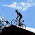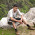## July 26, 2012

### Sample Problem on Setup and Hold

In the post Timing: Basics, we discussed about the basics of setup and hold times. Why is it necessary to meet the setup and hold timing requirements. And how frequency affects setup but does not affect hold.

Let us understand the concept with an example:

I hope the above waveforms are self explanatory.
Setup Slack in the above case (as inferred from the waveforms as well) is:

Setup Slack = Tclk - T(clk-2-q) - Tdata - T(su,FF2)

If this setup slack is positive, we say that setup time constraint is met. Note that setup slack depends upon the clock period and hence in turn frequency at which your design is clocked.

Let us consider hold timing:
Hold Slack = Tdata + T(clk-2-q) - T(ho,FF2)

As evident from the above equation, hold slack is independent of the frequency of the design.

Note:
• Setup is the next cycle check, we would take the setup time T(su,FF2) of FF2 into account while finding setup slack at input pin of FF2.
• Hold time is the same cycle check, we would take the hold time T(ho,FF2) of FF2 into account while computing the hold slack at input pin of FF2.
Try and grasp this example. I shall introduce the concept of clock skew next.

#### 11 comments:

1.Thanks for good article. Please correct me if my understanding is wrong.

So for the hold, what are the launch and capture flops? or Hold doesn't have that concept?
Here, hold slack mentioned is for FF1?, so we should consider the Tdata(FF1) and Tclk-q(FF1) right?
So, if this value [ Tdata( FF1 ) + Tclk-q(FF1 ) - T-hold ] is negative, then we have a hold violation on FF1 correct?

Thanks,
Bond007

1.Hello!
Don't think about launch and capture from the view of setup and hold. Launch and Capture are the terms which signify the launching & capturing of data. So, irrespective of the fact whether we are analyzing hold or setup, launch and capture flops would be the same.
The hold slack mentioned here is for FF2. Note that: when we say: hold violation, it means that the flop in question is receiving the previous data.
If the above equation is not met, then the FF2 would receive the data (which might be metastable) in the very same cycle that it was actually supposed to capture in the next cycle.

Let me know if you didn't understand anything. I would encourage you to solve other puzzles as well. You would enjoy solving them.

And thanks for the kind words of appreciation! :)

2.Thanks for your reply.

So you given below equation for hold slack for FF2. Then we should use T(ho,FF2) in the equation right? or I'm still missing some point :(

Hold Slack = Tdata + T(clk-2-q) - T(ho,FF1).

Thanks,
Bond007

3.Yes! It should be FF2. You got it absolutely right. Apologies for the typo. Thanks for pointing it out.

4.when are you introducing Skews here? I'm looking forward to it.

Thanks,
Bond007

5.Hello. I have tried to cover it in a post. I hope you'd enjoy the post!
Here's the link: http://vlsi-soc.blogspot.in/2013/03/clock-skew-implication-on-timing.html

6."irrespective of the fact whether we are analyzing hold or setup, launch and capture flops would be the same" - what does this actually mean can you please elaborate

2.Hello Palindrome,

Thanks for your sharing your knowledge with us.

Do you have any plan for below topics.
1) Timing analysis for Latches
2) Timing analysis for Set and Reset pins to Latches.
3) Timing analysis for Asynchronous reset to flops

Thanks in advance,
=R

3.So you have assumed assumed T buf_1 and T buf_2 as equal above right?

1.Oh yes! Just to make the skew = 0, and problem simple! :)

4.please consider a case where my clock delay, data delay and clk2q delay for ff1 is zero, we just have setup and hold time for ff2, how would we analyse the case